# How to determine the wire cross section - methods for determining the cross section

One of the important and main parts of electrical wiring is conductive cables. In order for everything to work as it should, without short circuits and molten wires, it is important to choose the wiring so that it can withstand the voltage. Make sure that the wire is reliable, safe and meets the requirements of operation, you can calculate the cross-section of the wire. If the wire is insufficient in cross section, it may cause a short circuit and a fire in the house. Let's figure out how to determine the cross-section of the wire to avoid such consequences.

## Serious question

If you have an electrical wiring plan according to your plan, then first of all you need to decide what wire size is needed. When you calculate the planned load on the wire by current, it will be immediately clear what section is needed for normal operation. For this purpose, use the table below.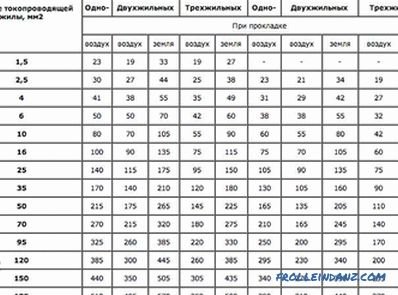Current selection

Suppose, having counted everything, you determined the current strength of 27 A. It turns out you need a two-wire cable, the cross section of which is 2.5 mm 2 . You went to the store, bought a good wire with a 2 section declared by the manufacturer with a cross section of 2.5 mm and installed electrical wires. After the time when the system was up and running, the automat on the dashboard is turned off. This may indicate a damaged line.

After you inspect the wiring, it may appear that there was a short circuit. The reason is simple - insulation on the wire melted due to strong heat. But how so? You did everything right, calculated, purchased the necessary wire. And the whole thing is not in the calculations and your connection error. The whole reason lies in deception. Yes, it is precisely in deception, because very often the manufacturers who produce wires deliver goods that do not correspond to the declared section. For example, you bought a cable with a wire area of ​​2.5 mm 2 , but when you checked it yourself, it turned out that the cable section is only 2.1 mm 2 , which is less than you need for power. So it turns out that the wire is heated, and increases the risk of a circuit.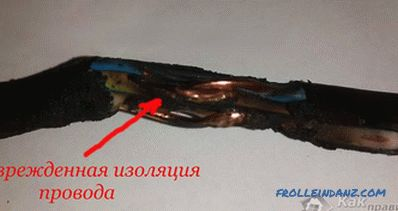Wire with damaged insulation

But why should cable companies do this? It's all about greed, because, by lowering the cross-section of the wire, the manufacturing company saves a fairly significant amount of money. Judge for yourself: to produce 1000 meters of wires with a cross section of 2.5 mm 2 , you will need approximately 22.3 kg of copper. But if you make them with a smaller section, 2.1 mm 2 , then their production will require 18.8 kg. It turns out that the savings in favor of the manufacturer is 3.5 kg of copper. And when large volumes of products are produced, this figure grows significantly.

It turns out that the definition of the wire cross section is an important and serious step that needs to be done when installing the wiring. This way you can avoid melting the insulation, the circuit and, as a result, the fire.

## How can you determine the cable cross-section

So you bought the wire, let's find out how it can be measured. For this purpose you will need:

• calipers;
• calculator;
• cable itself;
• stationery knife or stripper to remove the insulation.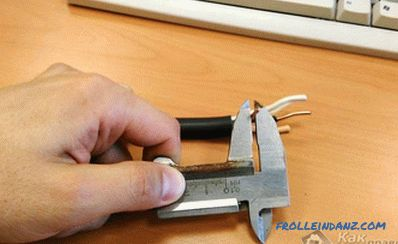Measuring with calipers

The section is determined by the diameter of the wire. Let's look at how to do this in stages.

1. Take the wire and remove the insulation from it with a stripper or clerical knife.
2. Using a calliper, measure the diameter of the wire.
3. Now, you have to remember the lessons of geometry at school. In order to determine the area of ​​a circle, there is a certain formula:

S cr = π r 2 ,

where the number π = 3, 14, and r is the radius of the wire.

But here we have a problem: using a caliper to measure the radius is impossible - only the diameter. Therefore, the formula needs to be slightly changed to the diameter. As we know, the radius is half the diameter. In order for our data to fit, the formula needs to be altered as follows:

S cr = (π d 2 ) / 4,

where d is the diameter of the wire .

In this case, the formula can be reduced by dividing the number π by four. As a result, we reach the standard formula for calculating the cross-section of the core by diameter:

Sc = 0.785d 2

It remains only to substitute the numbers to the formula and get the area of ​​the circle, which will be its cross section. For example, the diameter of your copper wire is 1.68 mm. This squared number is 2.8224. Multiply 2.8224 by 0.785. If to round off, then as a result, the desired section turned out to be 2.2 mm 2 .

That's all, as you can see, this procedure does not take much time and effort, but it is a very important part of the installation of electricity. After all, even a deviation of several tenths of a millimeter can play a cruel joke.But what if you are not an electrician and you do not have calipers at hand? Do not rush to run to the store, there is a simple method for calculating the diameter with the help of available tools.

## A simple method for determining the diameter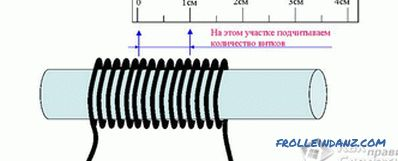The method for determining the cross-section

All you need is everyone has it at hand. This method will require only a pencil or pen and a ruler.

1. First of all, take the wire, the cross section of which you need to know, and remove 300-400 mm of insulation along it.
2. Take a pencil, pen, felt-tip pen, or whatever is on hand, and wind a wire around it. In order to achieve the most accurate result, the coils must be fitted close to each other.
The more turns you make, the more accurate the result will be. The recommended number is 10 or more turns.
3. Then count the number of turns you wound on the pencil, and write down this number or remember.
4. Using the ruler, measure the total length of the counted turns. For example, you got 19 turns, which are 32 mm long.
5. To determine the diameter of the wire, it remains only to divide the length by the number of turns. It turns out like this: 32/19 = 1.68 mm.

That's all, now it remains only to substitute the diameter of the wire in the formula and you will get a wire section of 2.2 mm 2 . This result can be considered accurate under two conditions: the coils were wound closely, and their number exceeds 10 pieces - the more, the better. As a result, you do not have to spend extra money.

The only disadvantage of this method: if you lived with a large cross section, you will not be able to wind it on a pencil.

## How to measure the cross-section if the wire is multicore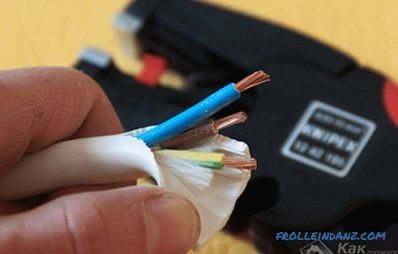Multicore multi-wire cable

Well, you say, if there is only one wire, it is easy to calculate the cross section. And what if it is stranded? There are a lot of such wires there, how to recognize it in such a case? Determining the cross-section of stranded wire is not as difficult as it seems. All you need to determine the cross section of one of the veins.

1. First, take the wire and remove the insulation from it.
2. Now all wiring needs to be disconnected from each other and recalculate their number.
3. Take one of the wires and measure its diameter.
4. Using the formula above, determine the area of ​​a single core.
5. Having a cross section of one core, you can find out the total cross section. To do this, the area of ​​one wire multiplied by their total. For example, you have a wire consisting of 15 wires. You have learned that the cross section of a single wire is 0.2 mm 2 . In order to find out their total cross section, 0.2 must be multiplied by 15. We get: 0.2 * 15 = 3 mm 2 .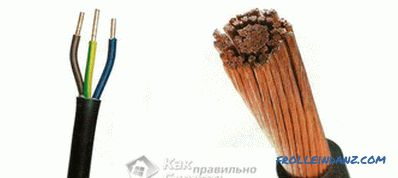Difference between multicore and multiwire cable

But it should be noted that all the wires in The wire can not be connected closely. Due to the fact that they are round, an air gap is formed between them. It must be taken into account. To do this, multiply the resulting result by 0.91. Suppose, if the wire cross section was 3 mm 2 , then, multiplying it by a factor of 0.91, we obtain a cross section of 2.7 mm 2 . That's all. There is nothing difficult, you just need to follow the instructions.

As you can see, everyone who knows how to use formulas can determine the wire section. But, despite the simplicity of such work, it is one of the most important steps that must be done before wiring throughout the house.